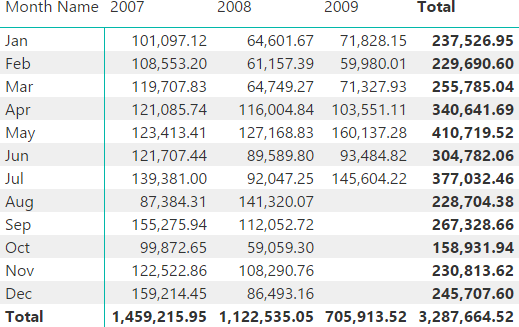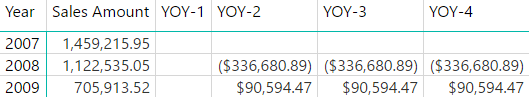The CALCULATE and CALCULATETABLE function in DAX transform existing row contexts into an equivalent filter context. This transformation is called context transition. A complete description is available in the article, Understanding Context Transition.

## Context Transition and Filter Arguments

The interaction between the filter arguments of CALCULATE or CALCULATETABLE and the filter context generated by the context transition is a possible source of confusion. For example, consider the following DAX expression that should compute the year over year of Sales Amount considering only the months present in both years. This is important for a correct evaluation of 2009, which does not have data between August and December.The following formula iterates every month and computes the difference only for months that have values in both the current year, and in previous years. For educational purposes, the calculation does not use a time intelligence function – the reason for that is explained later in the article.

```YOY-1 :=
SUMX (
VALUES ( 'Date'[YearMonth] ),
VAR SalesCY = [Sales Amount]
VAR SalesPY =
CALCULATE (
[Sales Amount],
TREATAS (
{ SELECTEDVALUE ( 'Date'[YearMonth] ) - 12 },
'Date'[YearMonth]
),
ALL ( 'Date' )
)
RETURN IF ( SalesCY > 0 && SalesPY > 0, SalesCY - SalesPY )
)
```

On line 9 the SELECTEDVALUE function retrieves the YearMonth value in the current filter context. The intention was to retrieve the single value of YearMonth iterated by SUMX. The context transition performed by CALCULATE on line 6 actually transforms the row context over ‘Date'[YearMonth] into a filter context, but this only applies to the first argument of CALCULATE – which is the expression found on line 7. The context transition does not have any effect on the following arguments of CALCULATE, from line 8 to line 12.

The following screenshot shows that the YOY-1 measure always returns blank. The filter context still has all the months of the selected year for every cell – due to the multiple selection, SELECTEDVALUE returns blank. The other measures in the screenshot (YOY-2, YOY-3, and YOY-4) will be explained later and return the correct result.There are two possible workarounds for that. The first one is to enforce the context transition on the filter argument in CALCULATE using another CALCULATE or CALCULATETABLE function (see line 9).

```YOY-2 :=
SUMX (
VALUES ( 'Date'[YearMonth] ),
VAR SalesCY = [Sales Amount]
VAR SalesPY =
CALCULATE (
[Sales Amount],
TREATAS (
{ CALCULATE ( SELECTEDVALUE ( 'Date'[YearMonth] ) ) - 12 },
'Date'[YearMonth]
),
ALL ( 'Date' )
)
RETURN IF ( SalesCY > 0 && SalesPY > 0, SalesCY - SalesPY )
)
```

The second workaround is to use the existing row context on line 9. The filter argument of CALCULATE does not receive any context transition, so any row context is still available.

```YOY-3 :=
SUMX (
VALUES ( 'Date'[YearMonth] ),
VAR SalesCY = [Sales Amount]
VAR SalesPY =
CALCULATE (
[Sales Amount],
TREATAS (
{ 'Date'[YearMonth] - 12 },
'Date'[YearMonth]
),
ALL ( 'Date' )
)
RETURN IF ( SalesCY > 0 && SalesPY > 0, SalesCY - SalesPY )
)
```

The previous examples do not rely a time intelligence function, because using a time intelligence function could be misleading when trying to understand the effects of the context transition. In fact, the following measure works properly using SAMEPERIODLASTYEAR, without requiring an additional CALCULATE or CALCULATETABLE to perform the context transition.

```YOY-4 :=
SUMX (
VALUES ( 'Date'[YearMonth] ),
VAR SalesCY = [Sales Amount]
VAR SalesPY =
CALCULATE (
[Sales Amount],
SAMEPERIODLASTYEAR ( 'Date'[Date] )
)
RETURN IF ( SalesCY > 0 && SalesPY > 0, SalesCY - SalesPY )
)
```

When a time intelligence function in DAX receives a column reference as a parameter, that column reference is implicitly wrapped in a CALCULATETABLE/VALUES function. The CALCULATETABLE function performs a context transition if one or more row contexts are active. In other words, any time intelligence function such as

```    SAMEPERIODLASTYEAR ( 'Date'[Date] )
```

```    SAMEPERIODLASTYEAR ( CALCULATETABLE ( VALUES ( 'Date'[Date] ) ) )
```

This is the reason why LASTDATE – which is a time intelligence function – should never be used within a row context: MAX is a better choice in that case, as explained in the blog post Difference between LASTDATE and MAX for semi-additive measures in DAX.

## Understanding the Evaluation Order

This section provides a more detailed description of the evaluation order of the filter arguments in CALCULATE vs. the context transition.

Consider this function:

```CALCULATE (
<expr>,
<filter1>,
<filter2>,
ALL ( <TableOrColumns> )
)
```

This is the exact evaluation order:

1. Evaluating filter arguments in the current filter context
2. Performing the context transition
3. Applying the filter arguments evaluated in step 1

Another way of looking at this is to imagine the context transition as a separate operation independent from CALCULATE that can be saved into a variable (T), such as any other filter expression.

The following meta-expression corresponds to the previous CALCULATE operation split into several steps. The context transition applies to the <expr> expression of CALCULATE first. The result of the context transition can be manipulated by the other filters, which are evaluated in an independent way.

```VAR F1 = <filter1>
VAR F2 = <filter2>
VAR T = <filter from context transition>
RETURN
CALCULATE (
CALCULATE (
<expr>,
F1,
F2,
ALL ( <TableOrColumns> )
),
T
)
```

It is important to remember that the innermost CALCULATE function is evaluated after the outermost CALCULATE has applied the filter T to the evaluation context. More details about this are available in the article, Order of Evaluation in CALCULATE Parameters.

## Conclusion

The filter arguments of CALCULATE and CALCULATETABLE do not receive the effect of a context transition. Any row context available outside of CALCULATE and CALCULATETABLE is available to filter arguments. Understanding this behavior is important in order to avoid unexpected results with complex calculations made in the filter arguments of these functions.

CALCULATEContext transition

Evaluates an expression in a context modified by filters.

`CALCULATE ( <Expression> [, <Filter> [, <Filter> [, … ] ] ] )`

CALCULATETABLEContext transition

Evaluates a table expression in a context modified by filters.

`CALCULATETABLE ( <Table> [, <Filter> [, <Filter> [, … ] ] ] )`

SELECTEDVALUEReturns the value when there’s only one value in the specified column, otherwise returns the alternate result.

`SELECTEDVALUE ( <ColumnName> [, <AlternateResult>] )`

SUMXReturns the sum of an expression evaluated for each row in a table.

`SUMX ( <Table>, <Expression> )`

SAMEPERIODLASTYEARContext transition

Returns a set of dates in the current selection from the previous year.

`SAMEPERIODLASTYEAR ( <Dates> )`

LASTDATEContext transition

Returns last non blank date.

`LASTDATE ( <Dates> )`

MAXReturns the largest value in a column, or the larger value between two scalar expressions. Ignores logical values. Strings are compared according to alphabetical order.

`MAX ( <ColumnNameOrScalar1> [, <Scalar2>] )`